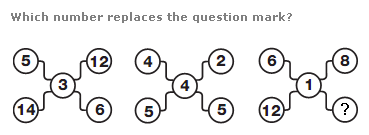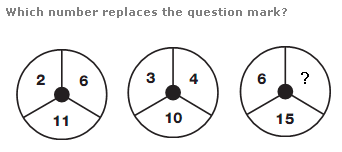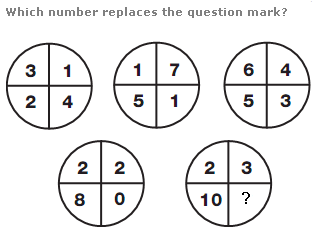# Puzzles - Number puzzles

### Exercise :: Number puzzlesAnswer : 3 Explanation : In each diagram, the sum of the top two and middle digits equals the sum of the lower 2 digits.Answer : 6 Explanation : In each circle, add together the left and right hand digits, and add 3 to give the value of the lower digit.Answer : 1 Explanation : tart with the top left circle, and move around the others in a W shape. The sum of the numbers in each circle follows the sequence of 10, 12, 14, 16 and 18.